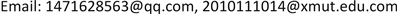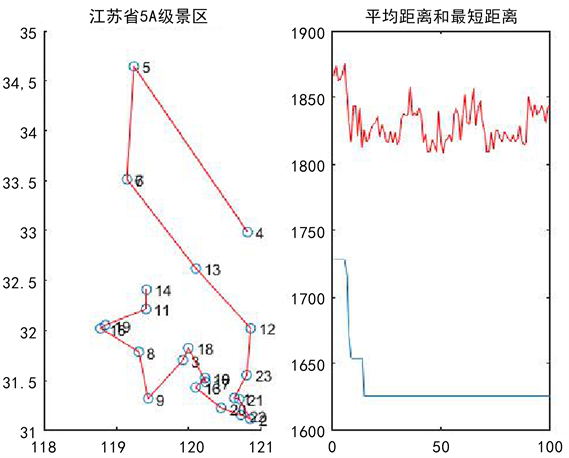﻿ 江苏省5A级景区旅游路线规划 5A Level Scenic Spot Tourism Route Planning of Jiangsu Province

Vol. 08  No. 05 ( 2019 ), Article ID: 30573 , 9 pages
10.12677/AAM.2019.85119

5A Level Scenic Spot Tourism Route Planning of Jiangsu Province

Lili Zheng, Qitong Ou

School of Applied Mathematics, Xiamen University of Technology, Xiamen FujianReceived: May 9th, 2019; accepted: May 24th, 2019; published: May 31st, 2019ABSTRACT

In this paper, the famous TSP problem is used to study the dynamic planning of tourism routes, a large number of literatures are referred in detail, and the data of 5A scenic spots in Jiangsu Province are collected and studied. The traffic data between the scenic spot and the city are determined, the complex traffic line network combined by high-speed rail and bus is constructed, and the shortest path model is established. At the same time, the ant colony algorithm is used to optimize and solve the model, and the ant colony algorithm is improved according to the results to obtain the shortest path planning model that meets the conditions, so as to provide route selection for more self-help travelers.

Keywords:Tourism Route Planning, Ant Colony Algorithm, The TSP Problem, Dynamic Programming Problem1. 引言

2. 相关研究综述

2.1. 江苏省5A级景区概述

2.2. 江苏省5A级景区的数据信息

Table 1. Tickets and accommodation fees for 5A scenic spots in Jiangsu Province

Table 2. The distance between some scenic spots

3. 旅游路线的数学模型规划

3.1. TSP模型问题

TSP问题  从描述上来看是在给定n个城市和各城市之间的距离的条件下，寻找一条遍历所有城市的最短路径，并且保证每个城市只被访问一次。针对江苏省的5A级景区的旅行路线规划，它的要求主要有：1、每一个景区仅游览一次；2、不含子巡回；3、整个旅游费最合理，4、旅游路线最短。

3.2. 模型假设

1) 假设任意两个风景区都有便捷的公路；

2) 不考虑浏览风景区的先后顺序；

3) 假设旅游费用不包括餐饮，不考虑其他省到达江苏省的费用；

4) 只考虑经济酒店，不考虑节假日带来的单位住宿费的提高；

5) 不区分高速公路和普通公路；

6) 假设在旅途中只有高铁或自驾两种交通工具，公交车的计费方式为路程乘以单位耗油费，并且在旅途中尽量以乘坐高铁为主，公交车为辅。

3.3. 符号说明

1) $scene=\left\{1,2,\cdots ,23\right\}$ 表示风景区集合，其中1代指出发地；

2) ${d}_{ij}$ 表示风景区和风景区两地之间的距离；

3) ${p}_{ij}$ 为二值变量，表示路线中风景区j紧随取值1，否则为0；

4) PZ是乘坐过公交车程中的费用；

5) PG是旅游过程中乘坐高铁的票价；

6) $Qidia{n}_{i}$ 乘坐高铁的起始位置；

7) $Zhongdia{n}_{i}$ 乘坐高铁的终点位置；

8) ${P}_{n}$ 是公路上行驶的单位里程耗油费(0.6672元/公里)；

9) PL是对应城市等级的单位住宿费；

10) PM是对应旅游景区的门票费用；

11) $N{L}_{i,j}$ 对应城市的住宿费次数；

12) $D{T}_{i,j}$ 是第i次旅游路线中在普通公路或高数公路上行驶的里程；

3.4. 模型建立

3.4.1. 目标函数一：最短路径

$\mathrm{min}z=\sum _{i}^{n}\sum _{j}^{n}{d}_{ij}{p}_{ij},\text{\hspace{0.17em}}\text{\hspace{0.17em}}\text{s}\text{.t}\text{.}$ (3.1)

$\forall j\in scene:\sum _{i\in scene}{p}_{ij}=1$ (3.2)

$\forall i\in scene:\sum _{i\in scene}{p}_{ij}=1$ (3.3)

$\forall i,j\in scene:{p}_{ij}\in \left\{0,1\right\}$ (3.4)

${v}_{i}-{v}_{j}+np\le n-1,{v}_{i},{v}_{j}\ge 0,i=1,2,\cdots ,n,j=2,3,\cdots ,n且i\ne j$ (3.5)

${v}_{i}\le n-1,{v}_{i}取整数$ (3.6)

${v}_{4}-{v}_{5}+n\le n-1$

${v}_{5}-{v}_{4}+n\le n-1$

3.4.2. 目标函数二：预算费用

${P}_{总}=\sum _{i=1}^{3}{P}_{i}$ (3.7)

${P}_{1}=\sum _{i,j\in scene}^{n}PG\left(Qidia{n}_{i},Zhongdia{n}_{j}\right)$ (3.8)

${P}_{2}=\sum _{i,j}^{n}{d}_{ij}×{P}_{n}$ (3.9)

${P}_{3}=\sum _{i\in scene}PM+\sum _{j\in scene}PL×N{L}_{i,j}$ (3.10)

4. 蚂蚁算法解决TSP模型

4.1. 蚁群算法原理

1) ${\alpha }_{ij}\left(t\right)$ 表示在t时刻，景区i到景区j间路径的信息强度。

2) ${\beta }_{ij}\left(t\right)$ 表示景区i和景区j的启发信息。一般情况下 ${\beta }_{ij}\left(t\right)=\frac{1}{{d}_{ij}}$

3) ${p}_{ij}^{s}\left(t\right)$ 表示在t时刻，蚂蚁s由景区i转移至景区j的概率。

${p}_{ij}^{s}\left(t\right)=\left\{\begin{array}{l}\frac{{\alpha }_{ij}^{k}\left(t\right){\beta }_{ij}^{l}\left(t\right)}{\sum _{r\in Tabl{e}_{s}}{\alpha }_{ir}^{k}\left(t\right){\beta }_{ir}^{l}\left(t\right)}\text{}j\in Tabl{e}_{s}\\ 0\text{}否则\end{array}$

$r=\left\{\begin{array}{l}\mathrm{arg}\mathrm{max}\left\{\left[\alpha \left(i,j\right)\right]{\left[\beta \left(i,j\right)\right]}^{l}\right\}\text{\hspace{0.17em}}\text{\hspace{0.17em}}\text{\hspace{0.17em}}\text{\hspace{0.17em}}\text{\hspace{0.17em}}\text{\hspace{0.17em}}若q\le {q}_{0}\\ R\text{\hspace{0.17em}}\text{\hspace{0.17em}}\text{\hspace{0.17em}}\text{\hspace{0.17em}}\text{\hspace{0.17em}}\text{\hspace{0.17em}}\text{\hspace{0.17em}}\text{\hspace{0.17em}}\text{\hspace{0.17em}}\text{\hspace{0.17em}}\text{\hspace{0.17em}}\text{\hspace{0.17em}}\text{\hspace{0.17em}}\text{\hspace{0.17em}}\text{\hspace{0.17em}}\text{\hspace{0.17em}}\text{\hspace{0.17em}}\text{\hspace{0.17em}}\text{\hspace{0.17em}}\text{\hspace{0.17em}}\text{\hspace{0.17em}}\text{\hspace{0.17em}}\text{\hspace{0.17em}}\text{\hspace{0.17em}}\text{\hspace{0.17em}}\text{\hspace{0.17em}}\text{\hspace{0.17em}}\text{\hspace{0.17em}}\text{\hspace{0.17em}}\text{\hspace{0.17em}}\text{\hspace{0.17em}}\text{\hspace{0.17em}}\text{\hspace{0.17em}}\text{\hspace{0.17em}}\text{\hspace{0.17em}}\text{\hspace{0.17em}}\text{\hspace{0.17em}}\text{\hspace{0.17em}}\text{\hspace{0.17em}}\text{\hspace{0.17em}}\text{\hspace{0.17em}}\text{\hspace{0.17em}}\text{\hspace{0.17em}}\text{\hspace{0.17em}}\text{\hspace{0.17em}}否则\end{array}$

${\alpha }_{ij}\left(t+1\right)=\left(1-p\right)\cdot {\alpha }_{ij}\left(t\right)+\Delta {\alpha }_{ij}$

$\Delta {\alpha }_{ij}=\sum _{k=1}^{m}\Delta {\alpha }_{ij}^{k}$

$\Delta {\alpha }_{ij}^{k}=\left\{\begin{array}{l}\frac{Q}{{L}_{k}},\text{\hspace{0.17em}}\text{\hspace{0.17em}}如果蚂蚁\text{ }k\text{ }在\text{ }t\text{ }时刻到t+1时刻通过i,j\\ 0,\text{\hspace{0.17em}}\text{\hspace{0.17em}}\text{\hspace{0.17em}}\text{\hspace{0.17em}}\text{\hspace{0.17em}}\text{\hspace{0.17em}}\text{\hspace{0.17em}}\text{\hspace{0.17em}}\text{\hspace{0.17em}}\text{\hspace{0.17em}}\text{\hspace{0.17em}}\text{\hspace{0.17em}}\text{\hspace{0.17em}}\text{\hspace{0.17em}}\text{\hspace{0.17em}}\text{\hspace{0.17em}}\text{\hspace{0.17em}}\text{\hspace{0.17em}}\text{\hspace{0.17em}}\text{\hspace{0.17em}}\text{\hspace{0.17em}}\text{\hspace{0.17em}}\text{\hspace{0.17em}}\text{\hspace{0.17em}}\text{\hspace{0.17em}}\text{\hspace{0.17em}}\text{\hspace{0.17em}}\text{\hspace{0.17em}}否则\end{array}$

4.2. 蚁群算法的实现步骤

1) $mc←0$${\alpha }_{ij}$$\Delta {\alpha }_{ij}$ 的初始化；将n只蚂蚁至于m个点上。

2) 将各蚂蚁的初始出发点置于当前解集中；对于每只蚂蚁k，按概率 ${p}_{ij}^{k}$ 移至下一项点j，将顶点j置于当前解集。

3) 计算各蚂蚁的路径长度，记录当前的最好解。

4) 按更新方程修改轨迹强度。

5) 对各边弧 $\left(i,j\right)$ ，置 $\Delta {\tau }_{ij}←0$$nc←nc+1$

6) 若小于预定的迭代次数且无退化行为，则转至步骤(2)。

7) 输出目前最好解。Figure 1. Operational results of ant colony algorithm

4.3. 改进蚁群算法的求解步骤

1) 初始化：迭代步数nc设置为0，在从大量的路径中选择比较优的路径，在这些路径留下信息素。将m只蚂蚁置于n个顶点上。

2) 根据当前位置调节各个值。

3) 将各蚂蚁的初始出发点置于当前解集中；对每只蚂蚁 $k\left(k=1,2,\cdots ,m\right)$ ，按照概率移至下一顶点j；将顶点j置于当前解集。

4) 对每只蚂蚁进行交叉变异操作，找出全局极值和全局极值位置。

5) 计算各蚂蚁的路径长度；记录当前的最好解。

6) 按更新方程修改轨迹强度。

7) 对各边弧 $\left(i,j\right)$ ，置 $\Delta {\tau }_{ij}←0$$nc←nc+1$

8) 若迭代步数小于预定的迭代次数且无退化行为(即找到的都是相同的解)，则转至步骤(2)。

9) 输出目前最好解。

4.3.1. 改进的蚁群算法的运行结果和分析Figure 2. Running results of improved ant colony algorithm

14 → 11 → 19 → 15 → 8 → 9 → 18 → 3 → 17 → 10 → 16 → 20 → 22 → 2 → 21 → 1 → 23 → 12 → 13 → 7 → 6 → 5 → 4。

4.3.2. 模型总结

5. 总结

5A Level Scenic Spot Tourism Route Planning of Jiangsu Province[J]. 应用数学进展, 2019, 08(05): 1042-1050. https://doi.org/10.12677/AAM.2019.85119

1. 1. 胡乔楠. 基于旅游文记的旅游景点推荐及行程路线规划系统[D]: [硕士学位论文]. 杭州: 浙江大学, 2015.

2. 2. 邹腊英. 基于TSP问题的旅游路线安排[J]. 兰州文理学院学报, 2015, 29(5): 23-25.

3. 3. 方昕. 一种新型启发PSO算法求解市区最优路径规划研究[J]. 计算机数与数字工程, 2018, 46(2): 270-275.

4. 4. 孙琼, 李林. 旅游路线规划蚁群算法的伪随机比例规则优化[J]. 科技通报, 2016, 32(1): 175-178.

5. 5. 袁光辉, 谢科, 邓林胜, 等. 旅游路线动态规划问题研究——以西安出发为例[J]. 数学的实践与认识, 2016, 46(15): 125-133.

6. 6. 赫标, 谭云兰, 王伟年, 等. 基于ACO的智能旅游景区路线规划设计[J], 井冈山大学学报(自然科学版), 2015, 36(1): 8-12.